# Sort/Rank Numeric Values with Duplicate Values Exist

Excel built-in RANK function can sort a set of values. If there are duplicate numbers, then the rank number is also duplicated. See the following example: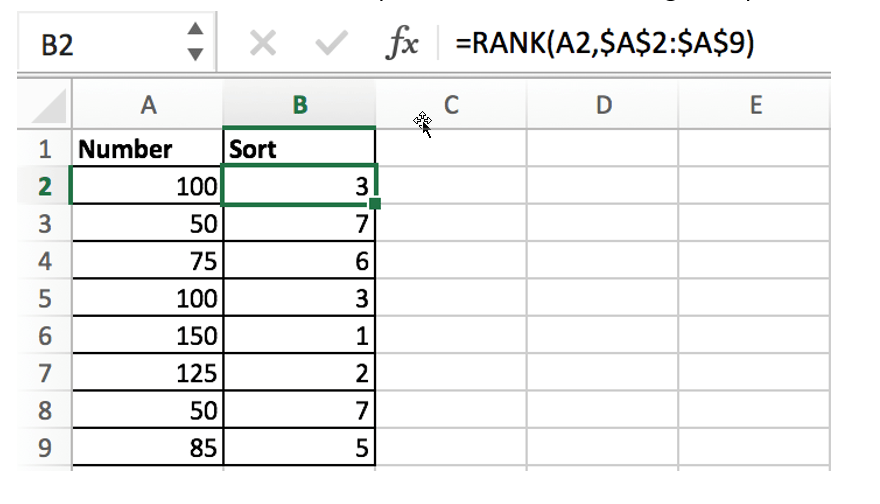There are two numbers “100” in range A2:A9, they are both the third largest number, so there are two “3” in the sort column and there is no number “4”, “4” is occupied by the second “3”.

If we want to avoid this problem (4 should be assigned to the second 100), when we encounter a duplicate number in a set of numbers, instead of assigning it the previously used rank number, we will assign it a new rank number that follows exactly the previous rank number.

See the new sequence: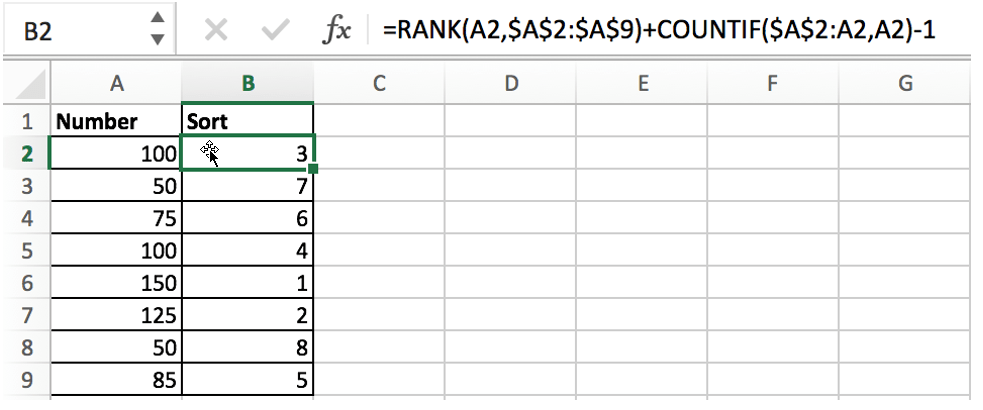## FORMULA

As we mentioned above, if you just apply the Excel RANK function to sort the numbers, once you encounter duplicate numbers, the duplicate rank number will be displayed.

When sorting numbers, we need to ensure below three points:

• Sorting numbers refer based on a proper order: ascending order or descending order
• That duplicate numbers are assigned to two numbers which are consecutive
• In Sort column, rank numbers are unique

In this example, the formula is:

`=RANK(A2,\$A\$2:\$A\$9)+COUNTIF(\$A\$2:A2,A2)-1`

Press Control+Shift+Enter to run this formula.

## EXPLANATION

We built this formula with the help of Excel RANK function and COUNTIF function, and also uses addition and subtraction operation.

### RANK EXPLANATION

The RANK function in Excel you can simply understand as rank a given set of values in ascending or descending order.

Syntax:

`=RANK(number, ref, [order])`
• number – rank this number
• ref – a set of numbers for ranking
• order – optional; ascending (1) or descending order (0)

# Ascending order means sorting the largest number as 1

# Descending order means sorting the smallest number as 1

You can set ascending or descending order according to your requirements. For example, in a race, you can set descending order when ranking time, but ascending order when ranking sales. In the example, order option is omitted, so the numbers are sorted in ascending order.

`=RANK(A2,\$A\$2:\$A\$9) – compare the number in cell A2 with the numbers in the range \$A\$2:\$A\$9`

In this example, the numbers are arranged in a fixed range. To avoid the range being adjusted when copying the formula, so we add \$ to lock the range. This formula works well if there are no duplicate numbers, and the RANK function will assign 1 to the largest number and 2 to the second largest number according to the rule, and so on.

Unfortunately, there are duplicate numbers in this example. To break the tie caused by the limitations of the RANK function, we added COUNTIF function to enhance the formula.

### COUNTIF EXPLANATION

Excel COUNTIF function returns the number of cells the meet one condition.

Syntax:

`=COUNTIF(range, criteria)`

In this example, the formula is:

`=COUNTIF(\$A\$2:A2,A2) – returns how many duplicate numbers in the selected range`

When copying the formula downward, the given range \$A\$2:A2 is extended, the starting cell A2 is locked, and the ending cell is the cell where the RANK function is currently working. Therefore, if we encounter a duplicate number in the range, the COUNTIF function will return the number of times the number is duplicated.

• If number only appears once, COUNTIF returns 1 as current row is included in selected range;
• If number appears twice, COUNTIF returns 2, and so on;
`=COUNTIF(\$A\$2:A2,A2)-1`

Since the number appears at least once in the selected range, for example, COUNTIF(\$A\$2:A2,A2) returns 1, we use the subtraction operation “-1” to offset the value returned by COUNTIF.

### FORMULA EXPLANATION

We take number “100” in cell A2 as an example, in B2 enter below formula:

`=RANK(A2,\$A\$2:\$A\$9)+COUNTIF(\$A\$2:A2,A2)-1`

Convert cell or range reference to actual number or array, the formula is:

`=RANK(100,{100;50;75;100;150;125;50;85})+COUNTIF(100,100)-1`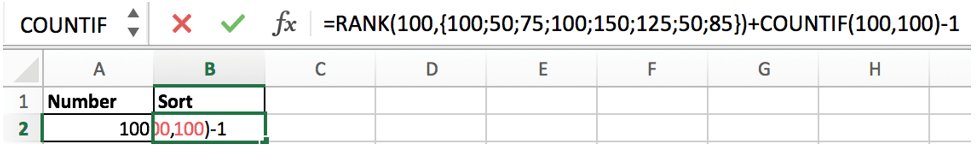As number “100” is the third largest number in the array, so “3” is returned by RANK function; COUNTIF function returns “1”, so the result is “3+1-1=3”.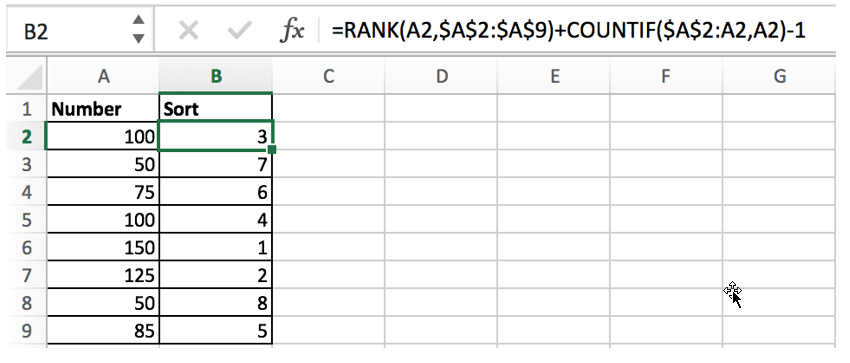We can see that the formula returns “4” for the same number “100” located in cell A5. This time COUNTIF function plays an important role and breaks tie perfectly.

In B5, the formula is:

`=RANK(A5,\$A\$2:\$A\$9)+COUNTIF(\$A\$2:A5,A5)-1`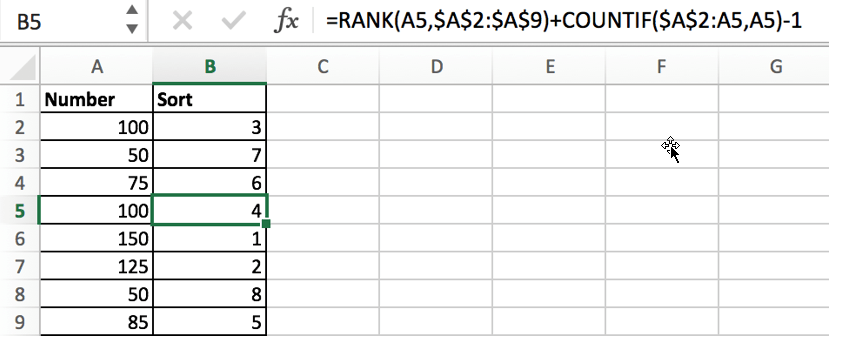RANK returns 3:

`-> 3+COUNTIF({100;50;75;100},100)-1`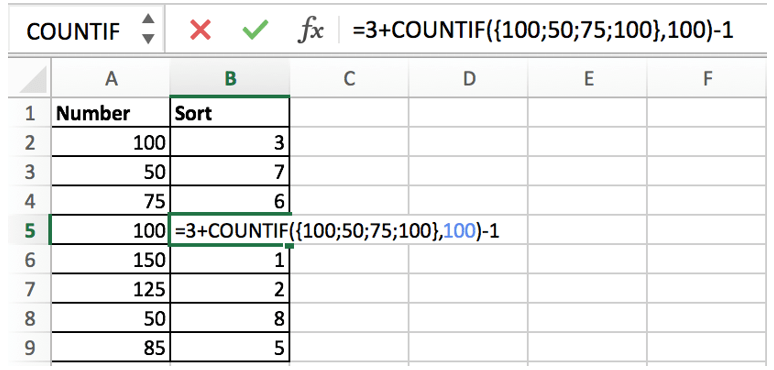COUNTIF returns 2 because {100;50;75;100} contains two “100”:

`-> 3+2-1 -> 4`

### Related Functions

• Excel COUNTIF function
The Excel COUNTIF function will count the number of cells in a range that meet a given criteria. This function can be used to count the different kinds of cells with number, date, text values, blank, non-blanks, or containing specific characters.etc.= COUNTIF (range, criteria)…
• Excel Rank function
The Excel RANK function returns the rank of a given number in a supplied range of cells.  If there are duplicated values in a list of values, it will be set the same rank.The syntax of the RANK function is as below:= RANK (number,ref,[order])….